# What Makes Ellipses… Ellipses?

Last time we used wild properties of ellipses to build some really easy—and some really devilish—golf courses. Specifically, I claimed that every ellipse has two magical points F1 and F2 (called foci) such that a ray from F1 always bounces off the ellipse and lands precisely at F2, and furthermore, this path always has the same length. Why does this happen? And how do we find these foci?

Let’s focus(!) on the last question first. Recall that an ellipse is a stretched circle. In other words, an ellipse is what forms when you slice a tall, circular tube (cylinder) along a slant: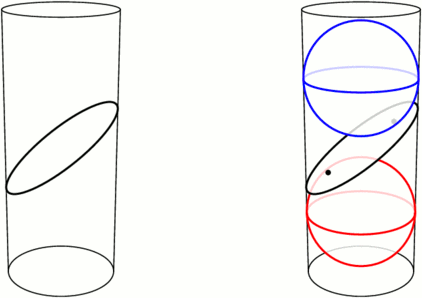Left: An ellipse is formed by slicing a cylinder. Right: Fitting spheres above and below the cut locates the ellipse’s foci.

Take a sphere that snugly fits inside the tube, and drop it down until it touches the ellipse-slice at a single point F1. Do the same with a sphere underneath, touching the slice at F2. These points turn out to be the foci of the ellipse. Let’s see why.

We can use this tubular setup to answer one mystery from earlier: for any point X on the ellipse, the sum of distances of XF1 and XF2 is always the same! The proof lies in the following animation. Segments XF1 and XA have the same length because they’re both tangent to the upper sphere from X, and similarly, XF2=XB. So the sum XF1+XF2 is just the length of segment AB, the height between the spheres’ equators.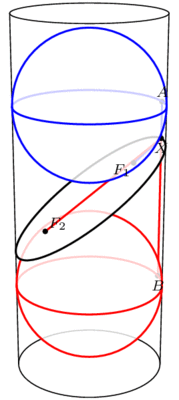The sum F1X+XF2 equals the length of AB and therefore does not change when X moves.

This has two neat consequences. First, it provides an elementary method for drawing ellipses (in real life!): all you need are two push pins and a loop of string, as illustrated below. The string ensures that the sum XF1+XF2 stays fixed while you trace the pen around, as long as you’re careful to keep the string taut throughout.

Second, what happens if we slice a cone instead of a cylinder? Perhaps surprisingly, we still get an ellipse! Indeed, as above, we can create a sphere on either side of the slice that snugly fits against the slice and the walls of the cone (the so-called Dandelin spheres), and exactly the same proof shows that XF1+XF2 stays constant as X moves around the edge.

But wait, there’s still an unanswered question! We’ve seen that the path F1X+XF2 has a fixed length, but why does light bounce off an ellipse along such a path? This is what we really cared about for mini-golf! Come back next time for the answer, and in the meantime, have a great 2 weeks.

# How Unilluminating!

You are standing in a large, oddly-shaped room, all of whose walls are lined with mirrors. Everything is dark except for a single light bulb somewhere else in the room. The light from this bulb spreads out in all directions and bounces off the mirrors, possibly many times over. Is it possible that, despite all the mirrors, you could still be standing in the dark, unable to see light from this bulb from any direction?

Said differently, consider the room as a large hole of mathematical mini-golf, where the locations of the ball (just one point) and cup (also just a point) are predetermined. Your goal is to pick a direction to hit the ball, hoping that it will reflect off the walls and eventually find its way to the cup. Is a hole-in-one always possible?When a light ray hits a wall, it bounces off in such a way that the angle of incidence (top left) equals the angle of reflection (top right). In other words, the dashed trajectory is reflected through the line of the mirror.

We assume that when a ray of light (i.e., the golf ball) hits a mirror, it reflects perfectly, as illustrated above. Let’s also assume that it never loses energy, so it will continue as far as necessary to reach its destination. There’s one corner case to consider, namely, what happens if the ray hits a corner of the room? Well, it’s (usually) ambiguous as to where it should reflect next, so let’s say that the ray just dies if it hits a corner. In the mini-golf formulation, our hole-in-one must avoid the hole’s corners.

With these rules in place, it turns out that it is possible to be left in the dark, or to make a hole-in-one impossible! One of the first examples, provided by Tokarski in 1995, is illustrated below; let’s call this room R.

Let’s see why this room R works, i.e., why point Q is not illuminated by a light source at P.

Notice that R is built from identical square cells. As our ray of light continues through the room, we can keep track of where it is in its current cell—but not which cell it is in—by imagining that the ray is just bouncing around in a single square, as illustrated below. Call this single square S. We are essentially folding up the light ray’s trajectory into S.An example light ray reflecting off the walls in Tokarski’s room, and the corresponding “folded” trajectory in a single cell (top middle). This ray gets very close to Q, but it will never reach it exactly. The grid is colored in a repeating pattern where each cell has 1 light yellow corner and three dark purple corners.

Color the grid points with light yellow and dark purple as illustrated above; in particular, P and Q are both yellow. Tokarski’s room has the interesting property that every dark purple point is in fact a corner of the room, so if the ray hits there, it dies. This means that the corresponding ray in square S would die when hitting one of the purple corners. So, in order for the light to reach Q in Tokarski’s room, the corresponding path in S must start at the yellow corner, end back at this same corner, and completely avoid the purple corners of the square. I claim this is impossible. Why?

Just as we folded room R into a single square, let’s consider unfolding square S to cover the whole plane. Because we’ve assumed perfect reflections, our trajectory in cell S unfolds to a straight line!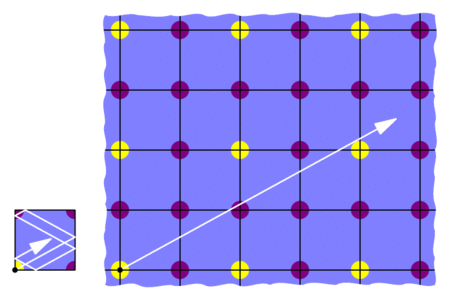“Unfolding” the the light’s trajectory in the square produces a straight path in an infinite grid of squares.

This means that we would need to find a straight trajectory from the initial yellow point to some other yellow point that does not hit any purple points. But this is impossible: all the yellows are blocked by purples! So the light can’t get from P to Q in Tokarski’s room, as claimed.

This room R is not the only example of an unilluminable room; with similar methods, many such rooms may be constructed. For example, in an effort to find an unilluminable room with the smallest number of edges, Tokarski provided the 26-sided polygon below on the left, which was then improved by Castro to a 24-sided polygon on the right. Both are built from 45-45-90 triangles instead of square cells.

Tokarski also provides the gem below (with no right angles!), and his paper gives recipes for a wide variety of other shapes.Another of Tokarski’s unilluminable rooms, this time with no right angles. The basic unit here is a triangle with angles 9, 72, and 99 degrees.

Here’s a challenge: so far, we’ve seen that it is possible to design a mathematical mini-golf hole that requires at least 2 shots. Can we make an even harder hole? Can we design one that needs at least, say, 10 shots? We’ll discuss this next time. See you then!

### Notes

1. George W. Tokarsky. Polygonal Rooms Not Illuminable from Every Point. American Mathathematical Monthly, 102:867–879, 1995. []
2. Here’s a proof: given any yellow-yellow segment, its midpoint lies on the grid. If this midpoint is purple, then we’re done. Otherwise, take half of the original segment and repeat this argument. []
3. D. Castro. Corrections. Quantum 7:42, 1997. []
4. It is not known whether 24 is minimal. []

# Three-Cornered Deltoids

[This is the third and final post in this series on triangle geometry. See the previous posts on Morley’s theorem and the 9-point circle.]

For our final exploration in this series, let’s again begin with our triangle ABC and a point P on the circumcircle of the triangle, i.e., the circle through the three vertices. If we drop P directly onto the three lines of the triangle at right angles, then by coincidence, these three points lie on a single line, called the Simson line of point P.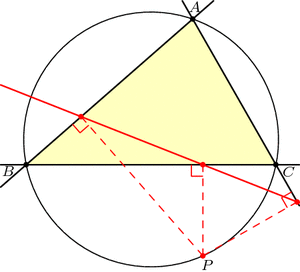Just for fun, let’s draw point Q diametrically opposite from P on the circumcircle, and let’s also look at Q‘s Simson line. How do these two Simson lines interact? Somewhat surprisingly, these lines intersect at right angles. The phenomenal part is that this point of intersection lies on the 9-point circle!Furthermore, as P and Q move around the circumcircle, the intersection of their Simson lines moves around the 9-point circle at twice the speed and in the opposite direction: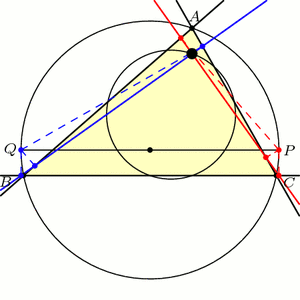This story gets even more unbelievable when we look at how the Simson lines move in this animation. As it turns out, the Simson lines trace a curve in the shape of a deltoid, which is like an equilateral triangle with curved sides. The deltoid traced here is called Steiner’s deltoid.And finally, here’s an incredible fact that ties everything together: If we draw the equilateral triangle around this deltoid, then the edges are parallel to the edges of the equilateral Morley triangle(s).Holy Morley!

### Notes

1. Note that we may have to extend the lines beyond the triangle. []
2. Specifically, a deltoid is the shape that results when you roll a circle inside a circle three-times larger and trace the path of a single point. See Wikipedia:Deltoid_curve for more information. []

# Many Morley Triangles

Given my blog title, how have I gone this long without discussing triangle geometry? I will rectify this gross negligence in the next few weeks. Let’s begin with a seemingly impossible fact due to Frank Morley.

Start with a triangle ABC, with any shape you wish. Now cut its angles into three equal parts, and extend these angle trisectors until they meet in pairs as illustrated below, forming triangle PQR. Morley’s amazing theorem says that this Morley triangle, PQR, will always be equilateral!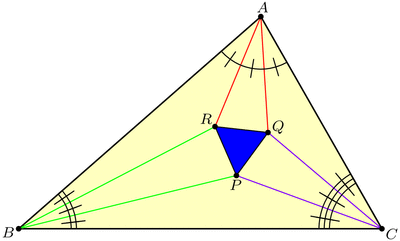Why would this be true? Well, Morley’s theorem tells us that this diagram has three nice 60-degree angles in the middle, but we may suspect that, in fact, all of the angles are nice! This key insight lets us piece together the following argument, where we build up the diagram backwards from its constituent pieces. Draw seven separate “puzzle piece” triangles with angles and side-lengths as shown below, where the original triangle ABC has angles $$3\alpha,3\beta,3\gamma$$ respectively. (Be sure to check that puzzle pieces with these specifications actually exist! Hint: use the Law of Sines.)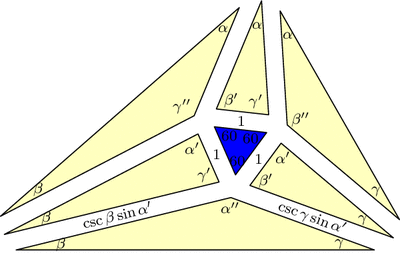Triangle ABC is built out of seven "puzzle pieces" with angles and side-lengths as specified here. In this diagram, $$\alpha^\prime=\alpha+60$$ and $$\alpha^{\prime\prime}=\alpha+120$$, and similarly for $$\beta$$ and $$\gamma$$.

Now, fit the pieces together: all matching edge-lengths are equal (by design), and the angles around vertex P add up to $$60+(\gamma+60)+(\alpha+120)+(\beta+60)=360$$ and similarly for Q and R, so the puzzle fits together into a triangle similar to our original triangle ABC. But now these pieces must make up the Morley configuration, and since we started with an equilateral in the middle, we’re done with the proof!

But there’s more to the Morley story. Let’s push A and C to make them swap positions, dragging the trisectors and Morley triangle along for the ride (a process known as extraversion). We end up using some external angle trisectors instead of only internal ones, but the Morley triangle remains equilateral throughout. This gives us a new equilateral Morley triangle for our original ABC!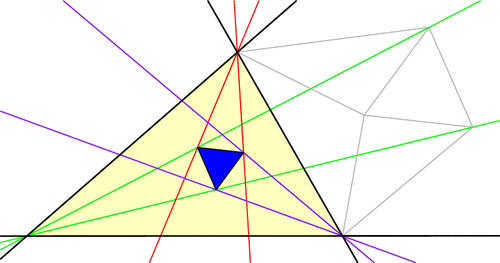In fact, each vertex of ABC has six angle trisectors (three pairs of two), and if you continue applying extraversions you’ll soon uncover that our original triangle ABC has 18 equilateral Morley triangles arranged in a stunning configuration! (Click for larger image.)Here’s a challenge: the diagram above has 27 equilateral triangles, but only 18 of them are Morley triangles (i.e., are made from a pair of trisectors from each vertex). Which ones are they?

### Notes

1. This is a slight modification of a proof by Conway and Doyle. []

# Seeing Stars

Let us now take a closer look at the hex-fractal we sliced last week. Chopping a level 0, 1, 2, and 3 Menger sponge through our slanted plane gives the following:

This suggests an iterative recipe to generate the hex-fractal. Any time we see a hexagon, chop it into six smaller hexagons and six triangles as illustrated below. Similarly, any time we see a triangle, chop it into a hexagon and three triangles like this:A recipe for constructing the hex-fractal: replace each hexagon with 6 hexagons and 6 triangles, and replace each triangle with 1 hexagon and 3 triangles.

In the limit, each triangle and hexagon in the above image becomes a hex-fractal or a tri-fractal, respectively. The final hex-fractal looks something like this (click for larger image):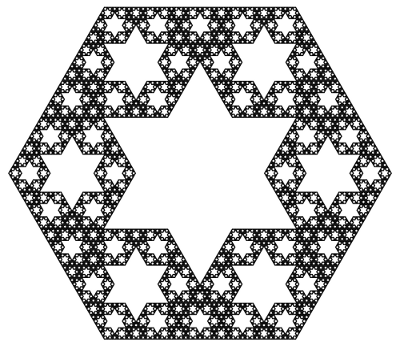Now we are in a position to answer last week’s question: how can we compute the Hausdorff dimension of the hex-fractal? Let d be its dimension. Like last week, our computation will proceed by trying to compute the “d-dimensional volume” of our shape. So, start with a “large” hex-fractal and tri-fractal, each of side-length 1, and let their d-dimensional volumes be h and t respectively.

Break these into “small” hex-fractals and tri-fractals of side-length 1/3, so these have volumes $$h/3^d$$ and $$t/3^d$$ respectively (this is how “d-dimenional stuff” scales). Since $$\begin{gather*}(\text{large hex}) = 6(\text{small hex})+6(\text{small tri}) \quad \text{and}\\ (\text{large tri}) = (\text{small hex})+3(\text{small tri}),\end{gather*}$$ we find that $$h=6h/3^d + 6t/3^d$$ and $$t=h/3^d+3t/3^d$$. Surprisingly, this is enough information to solve for the value of $$3^d$$. We find $$3^d = \frac{1}{2}(9+\sqrt{33})$$, so $$d=\log_3\left(\frac{9+\sqrt{33}}{2}\right) = 1.8184\ldots,$$ as claimed last week.

As a final thought, why did we choose to slice the Menger sponge on this plane? Why not any of the (infinitely many) others? Even if we only look at planes parallel to our chosen plane, a mesmerizing pattern emerges:

It takes a bit more work to turn the above computation of the hex-fractal’s dimension into a full proof, but there are a few ways to do it. Possible methods include mass distributions or similarity graphs.

This diagonal slice through the Menger sponge has been proposed as an exhibit at the Museum of Math. Sebastien Perez Duarte seems to have been the first to slice a Menger sponge in this way (see his rendering), and his animated cross section inspired my animation above.

### Notes

1. We’re assuming that the hex-fractal and tri-fractal have the same Hausdorff dimension. This is true, and it follows from the fact that a scaled version of each lives inside the other. []
2. There are actually two solutions, but the fact that h and t are both positive rules one out. []
3. Proposition 4.9 in: Kenneth Falconer. Fractal Geometry: Mathematical Foundations and Applications. John Wiley & Sons: New York, 1990. []
4. Section 6.6 in: Gerald Edgar. Measure, Topology, and Fractal Geometry (Second Edition). Springer: 2008. []

# Chess, Bored

[This is post #3 in a series on the Thue-Morse sequence, which was introduced two weeks ago and discussed further last week.]

While the Thue-Morse sequence appears in many branches of Mathematics, this sequence has also captured a place in the history of chess.

With only the basic rules of chess (specifically, how pieces move and capture and how a game may end by checkmate or stalemate), there is no guarantee that a game of chess will ever finish. For example, two (very bored!) players could theoretically move their knights back and forth ad infinitum without ever engaging each other or capturing a piece (but why would they want to?). A more realistic example is drawn below. In this game, black will soon checkmate white if given the freedom to move, and white has no way to win. However, white can avoid losing by keeping black in an unending runaround with repeated checks, as illustrated. In this case (a phenomenon known as perpetual check), forcing an infinite game is more advantageous for white than allowing an otherwise inevitable loss.

Because of this possibility of never finishing, a search began for new chess rules to guarantee that every game of chess eventually ends. One suggested rule was:

A chess game ends with a draw if a sequence of moves—with all pieces in exactly the same positions—is played three times successively.

The hope was that any sufficiently long chess game would eventually repeat itself enough to break this rule. However, as Mathematician and Chess Grandmaster Max Euwe showed in 1929, this hope is not correct. Indeed, there exist infinite chess games that never repeat the same block of moves three times in a row!

How did he prove this? With the Thue-Morse Sequence, of course! He proved the very unintuitive fact that the Thue-Morse sequence has no chunk repeated three times consecutively. Such a thrice-repeated sequence is called a cube, so this property can be restated by saying that the Thue-Morse sequence is cube-free. For example, you will never find the sequences 1 1 1 or 011 011 011 in the Thue-Morse sequence. (Challenge: show that these two sequences do not appear. Harder challenge: show that the Thue-Morse sequence is cube free!) So, if the “very bored” knight-flippers described above decide to move their right knights or left knights according to the 1s and 0s of the Thue-Morse sequence, the resulting game will have no tripled move sequences.

Luckily, the above rule is not part of the current official chess rule set. There are now two rules on the books that can be used to eventually end any chess game. (In fact, either one would suffice. [Prove this!]) A draw may be declared in either of these situations:

• The exact same board position appears three times ever during a game. (Every detail must be the same, such as whose turn it is, which kings have the ability to castle, etc.)
• No pawn is moved and no piece is captured in 50 consecutive turns. (A turn consists of one move by each player.)

However, there’s (always) a catch. These two rules only take effect if a player notices and decides to declare a draw. So even with all modern rules in effect, the bored knight-flippers are still allowed to play their infinite game of chess—cube-free or not.

### Notes

1. A great survey called The ubiquitous Prouhet-Thue-Morse sequence by Allouche and Shallit documents many sightings of this sequence throughout Mathematics, including Combinatorics, Differential Geometry, Number Theory, Analysis, and as discussed here, Combinatorial Game Theory. []
2. Manfred Börgens, Max Euwe’s sequence []
3. in his paper titled Mengentheoretische Betrachtungen über das Schachspiel []

# Thue-Morse Navigating Turtles

Here’s a simple rule to generate a pretty sequence of 1s and 0s: Start with the list 1 (just one digit) and repeatedly form a new list by flipping all the bits (1s become 0s and vice versa) and attaching this to the end: $$1 \to 10 \to 1001 \to 10010110 \to 1001011001101001 \to \cdots.$$ For example, to get from 1001 to the next sequence, we swap 1s and 0s to form 0110, and then we join these together to make 10010110. By continuing this process we can make our list longer and longer, and the limiting sequence $$1001011001101001011010011001011001101\cdots$$ is called the Thue-Morse Sequence.

What did I mean by calling this sequence “pretty”? Well, what does this sequence “look like”? One way to visualize it is with a turtle program. Imagine a turtle (let’s call him Leo) walking along the plane, and treat the digits of the Thue-Morse sequence as a list of instructions for the turtle as follows: When Leo sees the digit 1, he steps forward one foot; a 0 tells Leo to turn left by 60 degrees. What does Leo’s path look like? Here are the first 32 instructions he follows: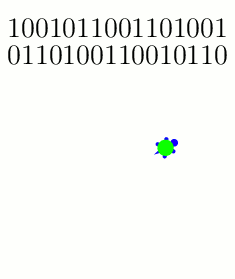The turtle follows these instructions on the first 32 entries of the Thue-Morse sequence: {1: Step; 0: Turn 60}.

And here are snapshots of his path as he gets farther into the Thue-Morse sequence:

Why did we pick these instructions? What if Leo turns 120 degrees instead of 60 degrees when he sees a 0?The turtle follows the Thue-Morse sequence with a slightly different set of instructions: {1: Step; 0: Turn 120}. Shown here after 2^6, 2^8, 2^10, and 2^12 instructions, respectively.

If you zoom out and/or squint a little, these paths are roughly following a famous fractal called the Koch curve. And indeed, the more steps you draw, the closer the resemblance will become (as analyzed in these two papers: [MH2005] and [KZH2008]). But why stop here? What happens if we change the turtle’s instructions even more? I played around with some new rule sets, and these too show how the Koch curve seems innately “programmed” into the structure of the Thue-Morse sequence:Three more pretty examples of the Thue-Morse sequence's "turtle power." In all three cases, the trajectories converge to the Koch curve. Left: {1: (Turn 180, Step); 0: (Turn 60, Step)}, after 2^8 instructions. Middle: {1: (Turn 180, Step); 0: (Turn 120, Step)}, after 2^10 instructions. Right: {1: Turn 180; 0: (Turn 15, Step)}, after 2^10 instructions.

Here’s a final challenge: if the Koch curve and the Thue-Morse sequence are so intimately related, shouldn’t we be able to find some turtle program instructions that trace the Koch curve without “squinting”? Specifically, the Koch curve is usually constructed as the limit of the following sequence of paths, where each iteration is made by stitching together four copies of the previous curve:The Koch curve is constructed as the limit of these paths, where each curve is formed from four (shrunken) copies of the previous curve.

Is there a set of turtle instructions whose path traces exactly these curves? In fact, there is! These instructions work: {1: (Step, Turn 60); 0: Turn 180}.

# Slicing Spheres

Last week we saw how to compute the area of a circle from first principles. What about spheres?

To compute the volume of a sphere, let’s show that a hemisphere (with radius $$r$$) has the same volume as the vase shown in the figure below, formed by carving a cone from the circular cylinder with radius and height $$r$$. Why this shape? Here’s why: if we cut these two solids at any height $$h$$ (between 0 and $$r$$), the areas of the two slices match. Indeed, the slice—usually called cross section—of the sphere is a circle of radius $$\sqrt{r^2-h^2}$$, which has area $$\pi(r^2-h^2)$$. Similarly, the vase’s cross section is a radius $$r$$ circle with a radius $$h$$ circle cut out, so its area is $$\pi r^2-\pi h^2$$, as claimed.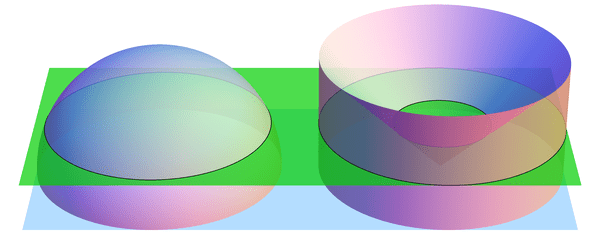When sliced by a horizontal plane at any height $$h$$, the hemisphere and vase have equal cross-sectional areas. (Shown here for $$h = 0.4\cdot r$$.) By Cavalieri's Principle, this implies that they have equal volumes.

If we imagine the hemisphere and vase as being made from lots of tiny grains of sand, then we just showed, intuitively, that the two solids have the same number of grains of sand in every layer. So there should be the same number of grains in total, i.e., the volumes should match. This intuition is exactly right:

Cavalieri’s Principle: any two shapes that have matching horizontal cross sectional areas also have the same volume.

So the volumes are indeed equal, and all that’s left is to compute the volume of the vase. But we can do this! Recall that the cone has volume $$\frac{1}{3} (\text{area of base}) (\text{height}) = \frac{1}{3}\pi r^3$$ (better yet, prove this too! Hint: use Cavalieri’s Principle again to compare to a triangular pyramid). Likewise, the cylinder has volume $$(\text{area of base}) (\text{height}) = \pi r^3$$, so the vase (and hemisphere) have volume $$\pi r^3 – \frac{1}{3} \pi r^3 = \frac{2}{3}\pi r^3$$. The volume of the whole sphere is thus $$\frac{4}{3}\pi r^3$$. Success!

The following visualization illustrates what we have shown, namely $$\text{hemisphere} + \text{cone} = \text{cylinder}.$$ The “grains of sand” in the hemisphere are being displaced horizontally by the stabbing cone, and at the end we have exactly filled the cylinder.